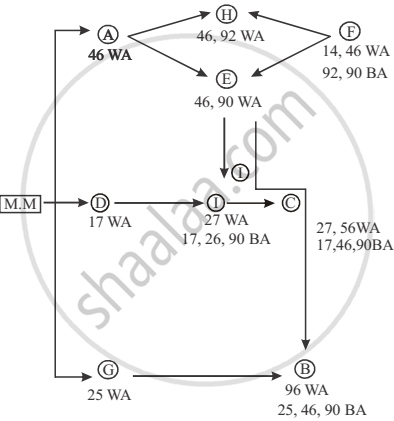# Which of the Following Statement is True? - Logical Reasoning

MCQ

Recently, the answers to a test held nationwide were leaked to a group of unscrupulous people. The investigative agency has arrested the mastermind and nine other people A, B, C. D, E, F, G, H and I in this matter. Interrogating them, the following facts have been obtained regarding their operation. Initially, the mastermind obtains the correct answer key. All the others create their answer key from one or two people who already possess the same. These people are called his/her "sources".

If the person has two sources, then he/she compares the answer keys obtained for both sources. If the key to a question from both sources is identical, it is copied, otherwise it is left blank.

If the person has only one source, He/she copies the source's answer into his/her copy. Finally, each person compulsorily replaces one of the answers (not a blank one) with a wrong answer in his/her answer key.

The paper contained 200 questions, so the investigative agency has ruled out the possibility of two or more of them introducing wrong answers to the same question. The investigative agency has a copy of the correct answer key and has tabulated the following data. This data represents question numbers.

 Name Wrong Answer(s) Blank Answer(s) A 45 - B 96 46,90,25 C 2756 17,46,90 D 17 - E 4690 - F 1446 92,90 G 25 - H 4692 - I 27 17,26,90

Which of the following statement is true?

#### Options

• C introduced the wrong answer to question 27

• E introduced the wrong answer to question 46.

• F introduced the wrong answer to question 14

• H introduced the wrong answer to question 46

#### Solution

F introduced the wrong answer to question 14

Explanation:

On the basis of given information, the following result can be obtained. Initially, mastermind obtained correct  answer key(1) The result shows that A, D and G obtained the answer key  from mastermind.
(2) E and H obtained the answer key from A.
(3) F obtained the answer key from both E and H.
(4) I obtained the answer key from both E and D.
(5) C obtained the answer key from I only.
(6) B obtained the answer key from E and G.
(1) A, D and G replaced the answers 46, 17 and 25 respectively.
(2) H replaced the answer 92.
(3) E replaced the answer 90.
(4) F replaced the answer 14.
(5) I replaced the answer 27.
(6) C replaced the answer 56.
(7) B replaced the answer 96.

F introduced the wrong answer to question 14 is correct.

Concept: Problem Solving Ability (Entrance Exam)
Is there an error in this question or solution?